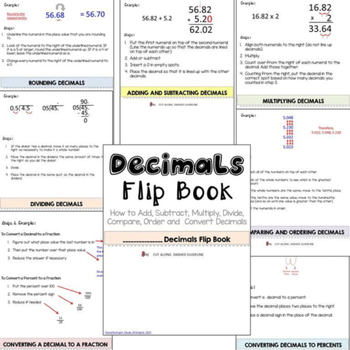# Math Flipbook Bundle - Math Resources for Teachers, Students and ParentsSubject
Resource Type
File Type
Zip (3 MB|106 pages)
\$15.75
Bundle
List Price:
\$17.50
You Save:
\$1.75
Products in this Bundle (5)
1. Volume Flip Book This Volume Flip Book will help students figure out how to find the volume of many different shapes with step by step directions and examples. No gluing! Just cut, stack and staple in less than two minutes! The Volume Flip Book has the following six sections: - Volume of a Cube
2. Surface Area Flip Book This Surface Area Flip Book will help students figure out how to find the surface area of many different 3D shapes and it is simple to assemble. No gluing! Just cut, stack and staple in less than two minutes! The Surface Area Flip Book has the following six sections: - Sur
3. Do your students struggle with the steps of adding, subtracting, multiplying, dividing, rounding, ordering and converting decimals? The Decimals Flip Book will take the frustrations out of teaching decimals and will serve as a "cheat sheet" for your students. This Decimals Flip Book resource will
4. Do your students struggle with the steps of adding fractions, subtracting fractions, reducing fractions, finding equivalent fractions, multiplying fractions, dividing fractions, ordering fractions or comparing fractions? The Fractions Flip Book will take the frustrations out of teaching fractions a
5. This Area Flip Book resource will help students figure out how to find the area of many different polygons and it is simple to assemble. No gluing! Just cut, stack and staple in less than two minutes! The Area Flip Book has the following six sections: - Area of a Square - Area of a Rectangle - A
Bundle Description

The Math Flip Book Bundle is a growing bundle of my popular math flip books. These resources will help your students compute fractions, decimals, area, surface area, and volume. The flip books are easy to assemble. No gluing! Just cut, stack and staple in less than two minutes!

The flip books in this bundle are discounted by 30% and include:

The Fractions Flip Book has the following six sections:

- Reducing Fractions

- Finding Equivalent Fractions

- Multiplying Fractions

- Dividing Fractions

- Ordering and Comparing Fractions

The Decimals Flip Book has the following seven sections:

- Rounding Decimals

- Multiplying Decimals

- Dividing Decimals

- Comparing & Ordering Decimals

- Converting a Decimal to a Fraction

- Converting a Decimal to a Percent

The Area Flip Book has the following six sections:

- Area of a Square

- Area of a Rectangle

- Area of a Triangle

- Area of a Parallelogram

- Area of a Trapezoid

- Area of a Circle

The Surface Area Flip Book has the following six sections:

- Surface Area of a Cube

- Surface Area of a Rectangular Prism

- Surface Area of a Rectangular Pyramid

- Surface Area of a Cylinder

- Surface Area of a Sphere

- Surface Area of a Cone

The Volume Flip Book has the following six sections:

- Volume of a Cube

- Volume of a Rectangular Prism

- Volume of a Cylinder

- Volume of a Cone

- Volume of a Pyramid

- Volume of a Sphere

There are two sets in each flipbook. The first set is the Teacher’s (Master) Copy that is pre-filled with examples and steps. The other is a Student Copy which is blank. There are two ways to use this resource:

PREFERRED WAY TO USE THESE RESOURCES

Teacher will have the Teacher’s (Master) Copy and students will have the blank Student Copy. As the teacher teaches each skill, the students will fill out their Student Copy to resemble the Teacher’s Master Copy. Students will keep this with them to use as a reference as they complete classwork and homework.

ANOTHER WAY TO USE THESE RESOURCES

Simply print out the Teacher (Master) Copy and give to students to use as a cheat sheet. Students will keep this with them to use as a reference as they complete classwork and homework.

-------------------------------------------------------------------------------------------

Do you want 50% of all my new uploads? Follow me!! All of my resources are 50% off in the first 24 hours of being uploaded. Follow me by clicking the green star under my name to be notified when I upload a new resource.

Total Pages
106 pages